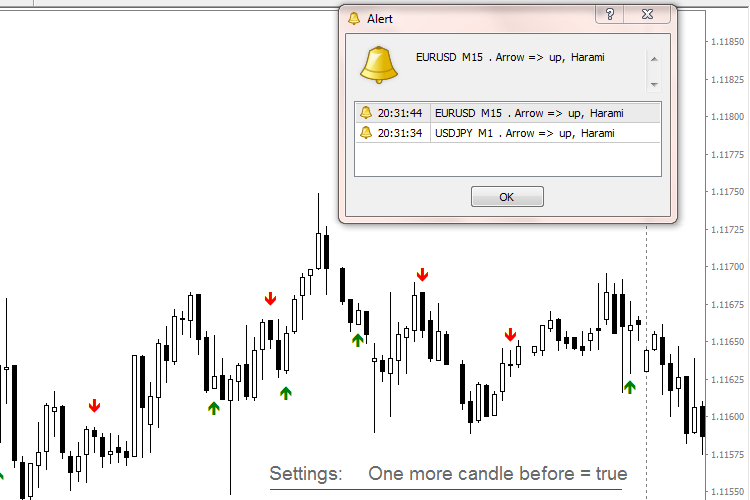# Harami MA RSI MACD – indicator for MetaTrader 4

I added a Candle to the Harami Pattern, the results in trend direction are convincing.

default-settings = “Harami” only

• MA and RSI and MACD are for experimental use
• all filters can be used alone or combined
• best results each for its own
• Timeframes: allRelated Posts

Input parameters

• barsToProcess=5000 – the number of periods of the chart, that are involved, if you use a M15 chart, one day only – 200 should be enough
• Alert_On – to get the alert on your screen
• MA_filter=false – false= the MA-settings are not active // true= the MA-settings are working
• RSI_filter=false – false= the RSI-settings are not active // true= the RSI-settings are working
• MACD_filter=false – false= the MACD-settings are not active // true= the MACD-settings are working
• One_Candle_before=false – false= the last candle before the pattern is not involved // true= the last candle before the pattern is involved and must have the same direction as the first pattern-candle
• Trend_MA=12
• MA_shift=0
• MA_Mode=Exponential
• MA_price=High price
• RSI_Period=5
• RSI_upper_level=70
• RSI_lower_level=30
• MACD_fast_EMA=12
• MACD_slow_EMA=26
• MACD_SMA=9